# Internal and external angles

Calculate the remaining internal and external angles of a triangle, if you know the internal angle γ (gamma) = 34 degrees and one external angle is 78 degrees and 40 '. Determine what kind of triangle it is from the size of its angles.

α =  101.3333 °
β =  44.6667 °
γ =  34 °
α' =  78.6667 °
β' =  135.3333 °
γ' =  146 °

### Step-by-step explanation:

$\alpha =180-\left(78+40\mathrm{/}60\right)=101.3333\text{°}=101\mathrm{°}2{0}^{\mathrm{\prime }}$
$\beta =180-\left(180-\left(78+40\mathrm{/}60\right)+34\right)=44.6667\text{°}=44\mathrm{°}4{0}^{\mathrm{\prime }}$
$\gamma =34=34\text{°}$
${\alpha }^{\mathrm{\prime }}=78+40\mathrm{/}60=78.6667\text{°}=78\mathrm{°}4{0}^{\mathrm{\prime }}$
${\beta }^{\mathrm{\prime }}=180-\left(78+40\mathrm{/}60\right)+34=135.3333\text{°}=135\mathrm{°}2{0}^{\mathrm{\prime }}$
${\gamma }^{\mathrm{\prime }}=180-34=146\text{°}$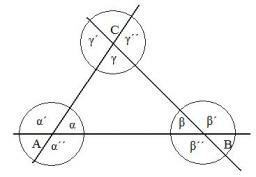Did you find an error or inaccuracy? Feel free to write us. Thank you!Tips to related online calculators

#### You need to know the following knowledge to solve this word math problem:

We encourage you to watch this tutorial video on this math problem:

## Related math problems and questions:

• In triangleIn triangle ABC, the magnitude of the internal angle gamma is equal to one-third of the angle alpha. The size of the angle beta is 80 degrees larger than the size of the gamma angle. Calculate the magnitudes of the interior angles of the triangle ABC.
• TrianglesFind out whether given sizes of the angles can be interior angles of a triangle: a) 23°10',84°30',72°20' b) 90°,41°33',48°37' c) 14°51',90°,75°49' d) 58°58',59°59',60°3'
• Internal anglesOne internal angle of the triangle JAR is 25 degrees. The difference is the size of the two others is 15°. Identify the size of these angles.
• Outer anglesThe outer angle of the triangle ABC at the A vertex is 71°40 ' outer angle at the vertex B is 136°50'. What size has the inner triangle angle at the vertex C?
• Alfa, beta, gamaIn the triangle ABC is the size of the internal angle BETA 8 degrees larger than the size of the internal angle ALFA and size of the internal angle GAMA is twice the size of the angle BETA. Determine the size of the interior angles of the triangle ABC.
• Angles in triangle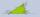Calculate the alpha angle in the triangle if beta is 61 degrees and 98 gamma degrees.
• Smallest internal angle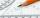Calculate what size has the smallest internal angle of the triangle if values of angles α:β:γ = 3:4:8
• Angles of the triangleABC is a triangle. The size of the angles alpha, beta are in a ratio 4: 7. The angle gamma is greater than the angle alpha by a quarter of a straight angle. Determine angles of the triangle ABC.
• Interior angles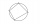In a quadrilateral ABCD, whose vertices lie on some circle, the angle at vertex A is 58 degrees and the angle at vertex B is 134 degrees. Calculate the sizes of the remaining interior angles.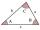The size of two internal angles of a triangle ABC are α=6/18π and β=7/18π. Calculate the size of the third angle.
• Angles ratioIn a triangle ABC, is true relationship c is less than b, and b is less than a. Internal angles of the triangle are in the ratio 5:4:9. The size of the internal angle beta is:
• Angles of a triangleIn triangle ABC, the angle beta is 15° greater than the angle alpha. The remaining angle is 30° greater than the sum of the angles alpha and beta. Calculate the angles of a triangle.
• The chordThe side of the triangle inscribed in a circle is a chord passing through the circle center. What size are the internal angles of a triangle if one of them is 40°?
• Triangle anglesIn a triangle, ABC, the interior angle at vertex C is twice the internal angle at point A. The outer angle at point B measured 117 degrees. How big is the external angle at vertex A?
• AnglesThe outer angle of the triangle ABC at the vertex A is 114°12'. The outer angle at the vertex B is 139°18'. What size is the internal angle at the vertex C?
• Angles in ratioThe size of the angles of the triangle are in ratio x: y = 7: 5 and the angle z is 42° lower than the angle y. Find size of the angles x, y, z.
• Angles in triangleThe triangle is ratio of the angles β:γ = 6:8. Angle α is 40° greater than β. What are the size of angles of the triangle?